# 3 DIFFERENTIATION RULES DIFFERENTIATION RULES If we are

• Slides: 383 DIFFERENTIATION RULESDIFFERENTIATION RULES If we are pumping air into a balloon, both the volume and the radius of the balloon are increasing and their rates of increase are related to each other. § However, it is much easier to measure directly the rate of increase of the volume than the rate of increase of the radius.DIFFERENTIATION RULES 3. 9 Related Rates In this section, we will learn: How to compute the rate of change of one quantity in terms of that of another quantity.RELATED RATES In a related-rates problem, the idea is to compute the rate of change of one quantity in terms of the rate of change of another quantity—which may be more easily measured. § The procedure is to find an equation that relates the two quantities and then use the Chain Rule to differentiate both sides with respect to time.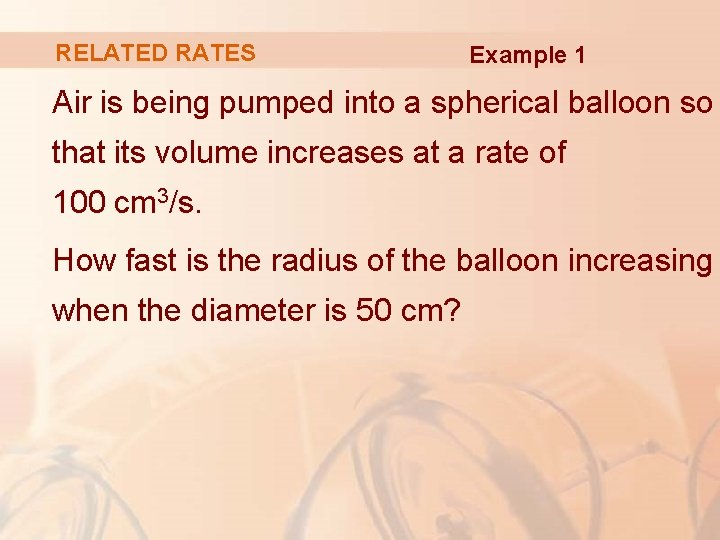RELATED RATES Example 1 Air is being pumped into a spherical balloon so that its volume increases at a rate of 100 cm 3/s. How fast is the radius of the balloon increasing when the diameter is 50 cm?RELATED RATES Example 1 We start by identifying two things: § Given information: The rate of increase of the volume of air is 100 cm 3/s. § Unknown: The rate of increase of the radius when the diameter is 50 cm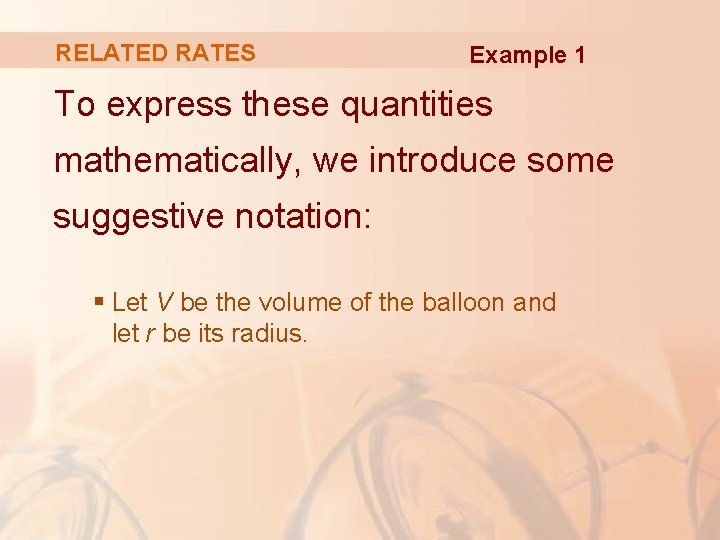RELATED RATES Example 1 To express these quantities mathematically, we introduce some suggestive notation: § Let V be the volume of the balloon and let r be its radius.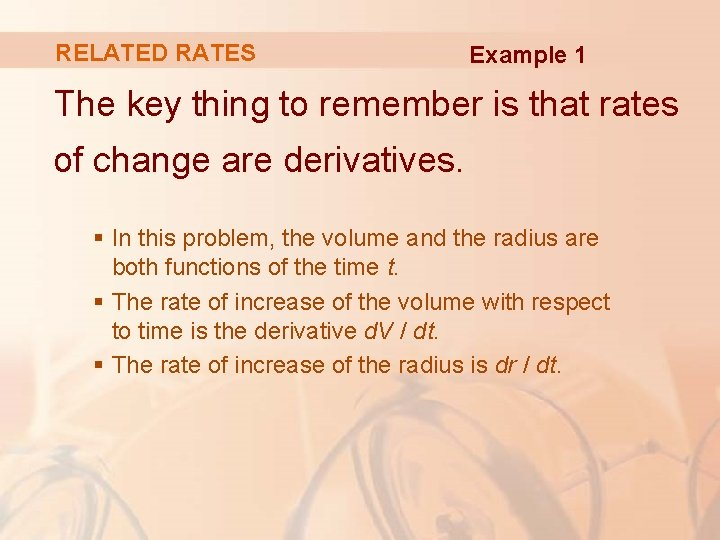RELATED RATES Example 1 The key thing to remember is that rates of change are derivatives. § In this problem, the volume and the radius are both functions of the time t. § The rate of increase of the volume with respect to time is the derivative d. V / dt. § The rate of increase of the radius is dr / dt.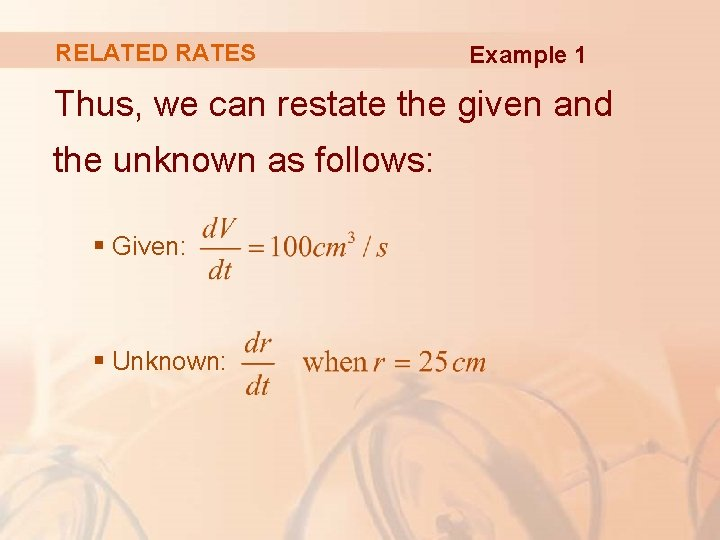RELATED RATES Example 1 Thus, we can restate the given and the unknown as follows: § Given: § Unknown: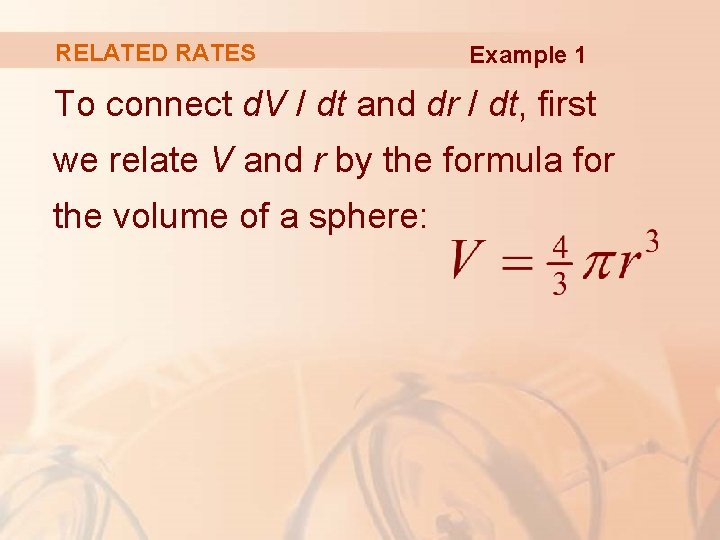RELATED RATES Example 1 To connect d. V / dt and dr / dt, first we relate V and r by the formula for the volume of a sphere: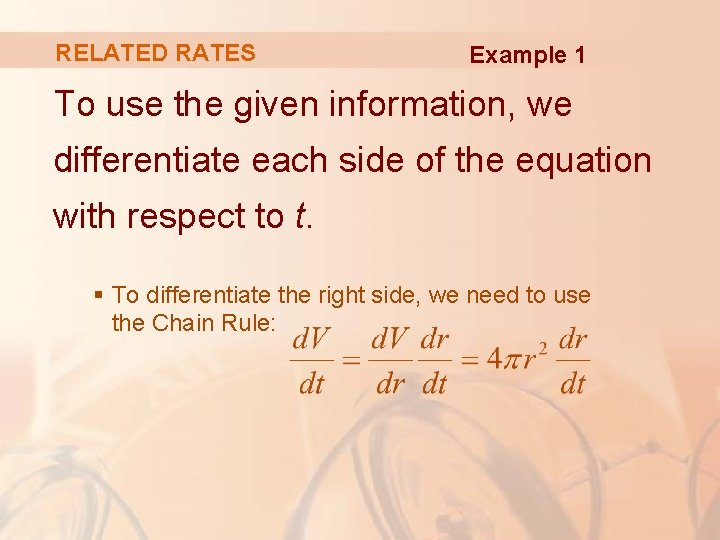RELATED RATES Example 1 To use the given information, we differentiate each side of the equation with respect to t. § To differentiate the right side, we need to use the Chain Rule: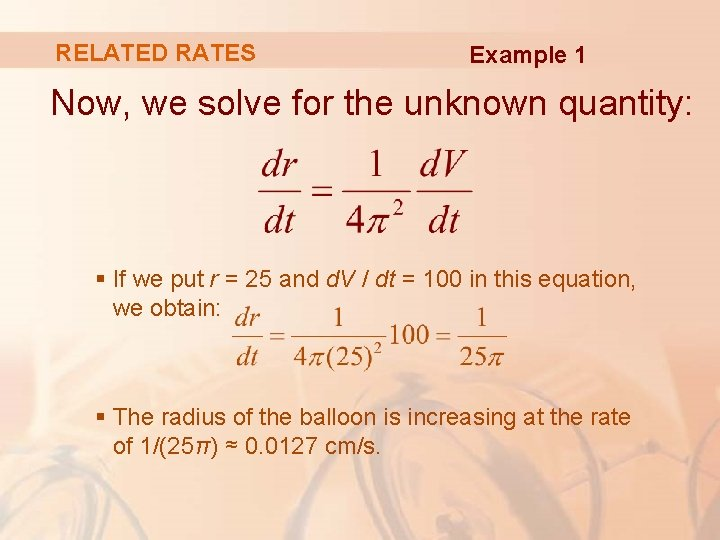RELATED RATES Example 1 Now, we solve for the unknown quantity: § If we put r = 25 and d. V / dt = 100 in this equation, we obtain: § The radius of the balloon is increasing at the rate of 1/(25π) ≈ 0. 0127 cm/s.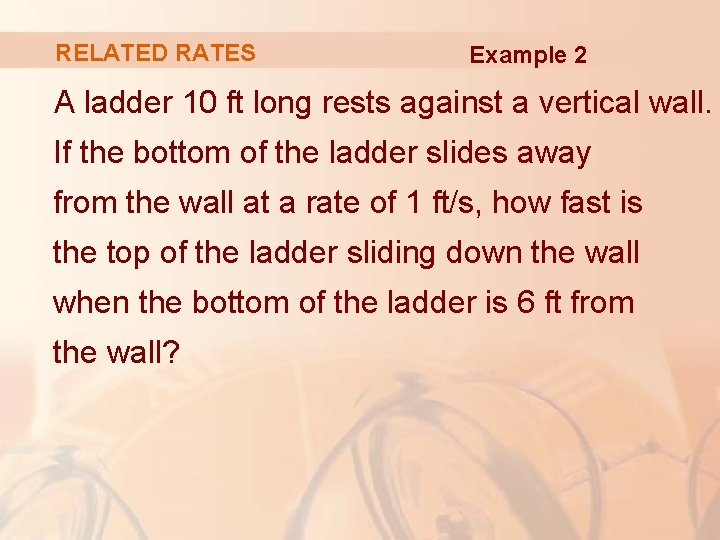RELATED RATES Example 2 A ladder 10 ft long rests against a vertical wall. If the bottom of the ladder slides away from the wall at a rate of 1 ft/s, how fast is the top of the ladder sliding down the wall when the bottom of the ladder is 6 ft from the wall?RELATED RATES Example 2 We first draw a diagram and label it as in the figure. § Let x feet be the distance from the bottom of the ladder to the wall and y feet the distance from the top of the ladder to the ground. § Note that x and y are both functions of t (time, measured in seconds).RELATED RATES Example 2 We are given that dx / dt = 1 ft/s and we are asked to find dy / dt when x = 6 ft.RELATED RATES Example 2 In this problem, the relationship between x and y is given by the Pythagorean Theorem: x 2 + y 2 = 100RELATED RATES Example 2 Differentiating each side with respect to t using the Chain Rule, we have: § Solving this equation for the desired rate, we obtain: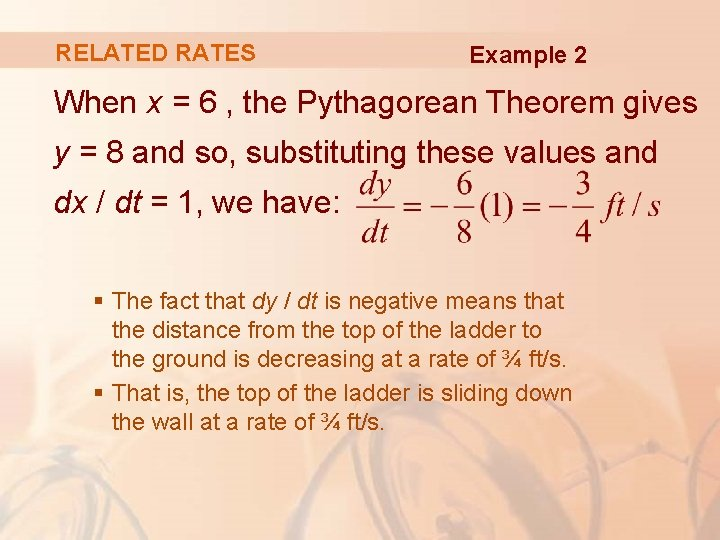RELATED RATES Example 2 When x = 6 , the Pythagorean Theorem gives y = 8 and so, substituting these values and dx / dt = 1, we have: § The fact that dy / dt is negative means that the distance from the top of the ladder to the ground is decreasing at a rate of ¾ ft/s. § That is, the top of the ladder is sliding down the wall at a rate of ¾ ft/s.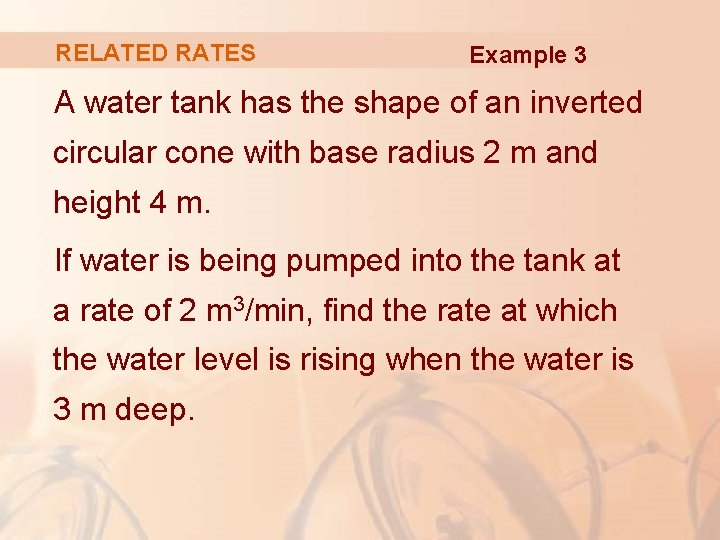RELATED RATES Example 3 A water tank has the shape of an inverted circular cone with base radius 2 m and height 4 m. If water is being pumped into the tank at a rate of 2 m 3/min, find the rate at which the water level is rising when the water is 3 m deep.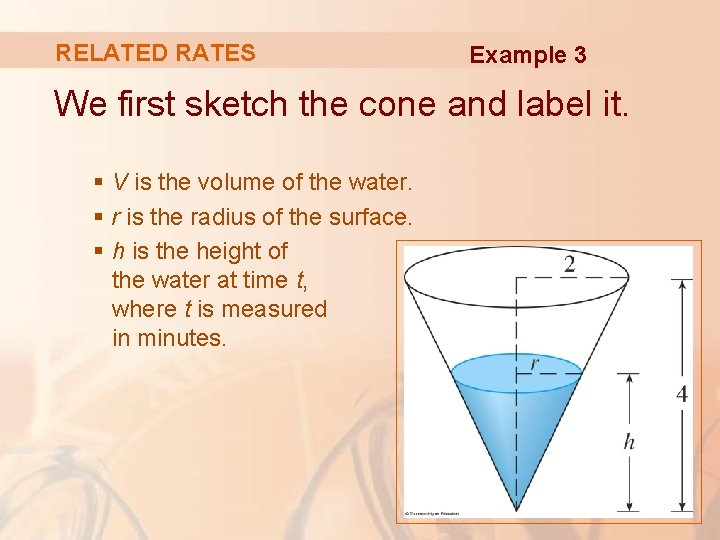RELATED RATES Example 3 We first sketch the cone and label it. § V is the volume of the water. § r is the radius of the surface. § h is the height of the water at time t, where t is measured in minutes.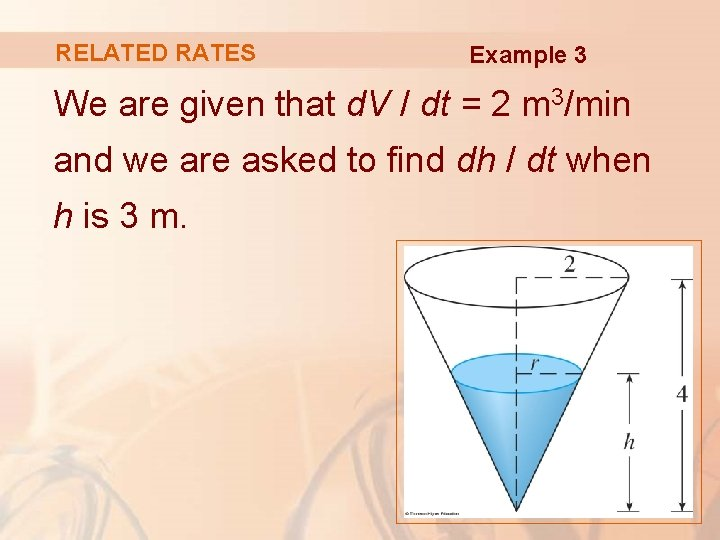RELATED RATES Example 3 We are given that d. V / dt = 2 m 3/min and we are asked to find dh / dt when h is 3 m.RELATED RATES Example 3 The quantities V and h are related by the equation § However, it is very useful to express V as a function of h alone.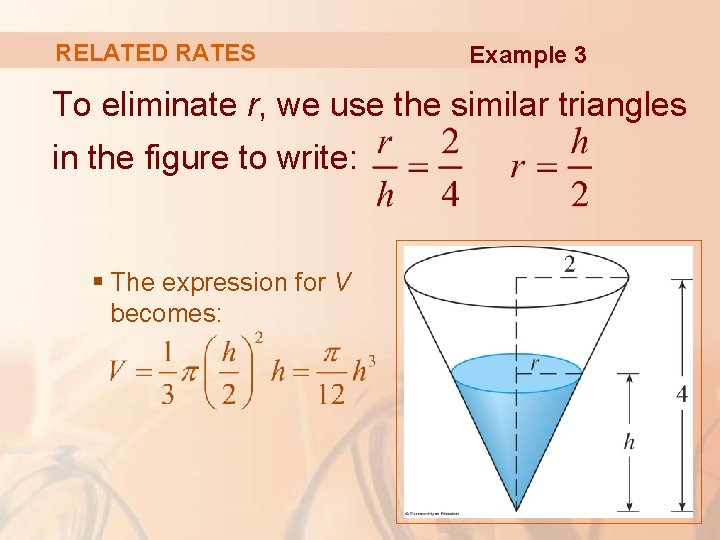RELATED RATES Example 3 To eliminate r, we use the similar triangles in the figure to write: § The expression for V becomes: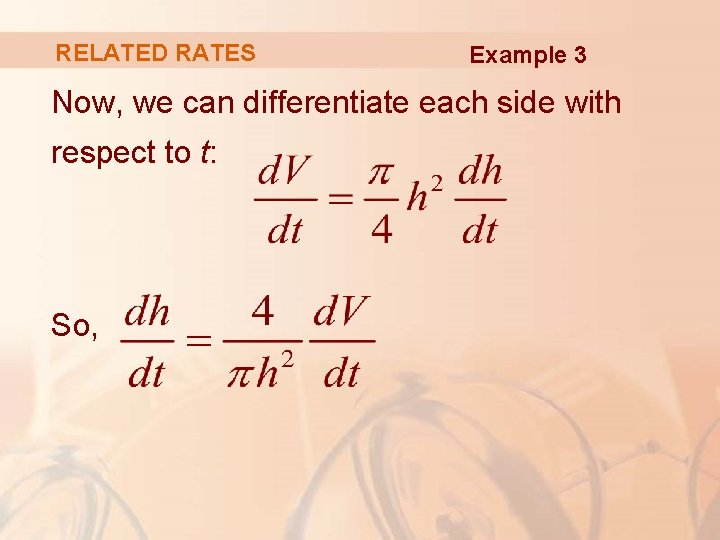RELATED RATES Example 3 Now, we can differentiate each side with respect to t: So,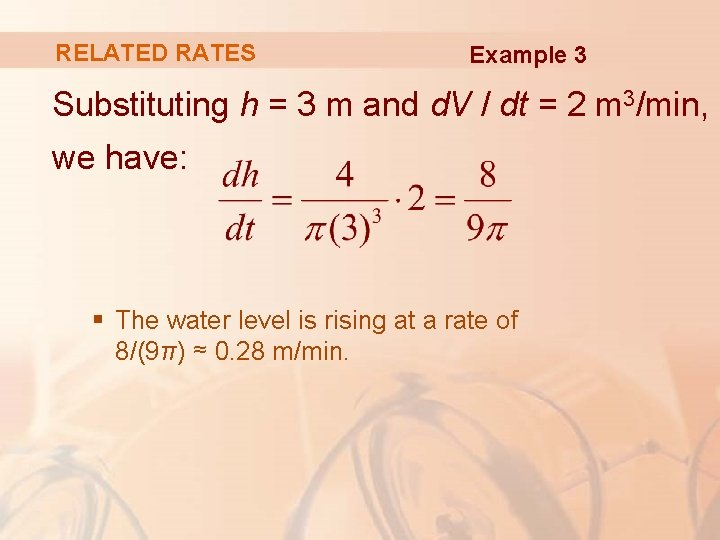RELATED RATES Example 3 Substituting h = 3 m and d. V / dt = 2 m 3/min, we have: § The water level is rising at a rate of 8/(9π) ≈ 0. 28 m/min.STRATEGY It is useful to recall some of the problemsolving principles from Chapter 1 and adapt them to related rates in light of our experience in Examples 1– 3. 1. Read the problem carefully. 2. Draw a diagram if possible. 3. Introduce notation. Assign symbols to all quantities that are functions of time.STRATEGY 4. Express the given information and the required rate in terms of derivatives. 5. Write an equation that relates the various quantities of the problem. If necessary, use the geometry of the situation to eliminate one of the variables by substitution (as in Example 3). 6. Use the Chain Rule to differentiate both sides of the equation with respect to t. 7. Substitute the given information into the resulting equation and solve for the unknown rate.STRATEGY The following examples are further illustrations of the strategy.RELATED RATES Example 4 Car A is traveling west at 50 mi/h and car B is traveling north at 60 mi/h. Both are headed for the intersection of the two roads. § At what rate are the cars approaching each other when car A is 0. 3 mi and car B is 0. 4 mi from the intersection?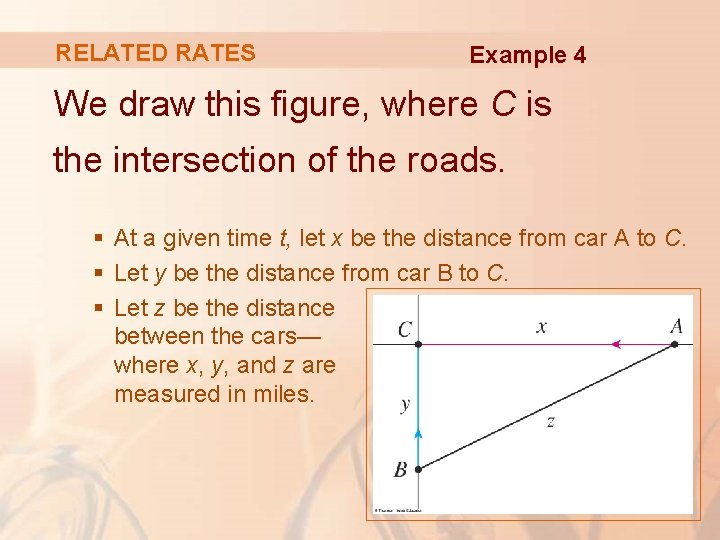RELATED RATES Example 4 We draw this figure, where C is the intersection of the roads. § At a given time t, let x be the distance from car A to C. § Let y be the distance from car B to C. § Let z be the distance between the cars— where x, y, and z are measured in miles.RELATED RATES Example 4 We are given that dx / dt = – 50 mi/h and dy / dt = – 60 mi/h. § The derivatives are negative because x and y are decreasing. We are asked to find dz / dt.RELATED RATES Example 4 The equation that relates x, y, and z is given by the Pythagorean Theorem: z 2 = x 2 + y 2 § Differentiating each side with respect to t, we have: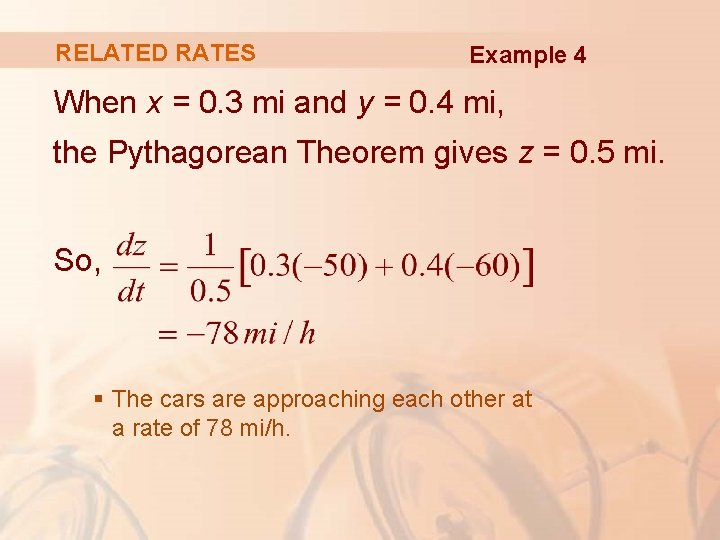RELATED RATES Example 4 When x = 0. 3 mi and y = 0. 4 mi, the Pythagorean Theorem gives z = 0. 5 mi. So, § The cars are approaching each other at a rate of 78 mi/h.RELATED RATES Example 5 A man walks along a straight path at a speed of 4 ft/s. A searchlight is located on the ground 20 ft from the path and is kept focused on the man. § At what rate is the searchlight rotating when the man is 15 ft from the point on the path closest to the searchlight?RELATED RATES Example 5 We draw this figure and let x be the distance from the man to the point on the path closest to the searchlight. § We let θ be the angle between the beam of the searchlight and the perpendicular to the path.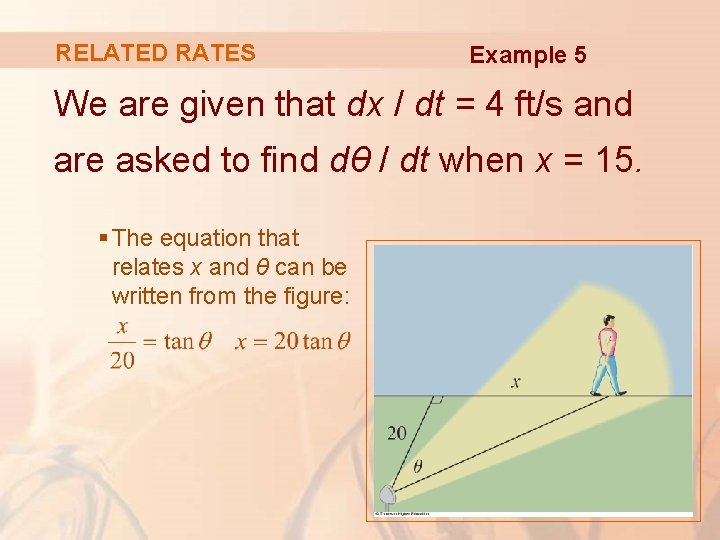RELATED RATES Example 5 We are given that dx / dt = 4 ft/s and are asked to find dθ / dt when x = 15. § The equation that relates x and θ can be written from the figure: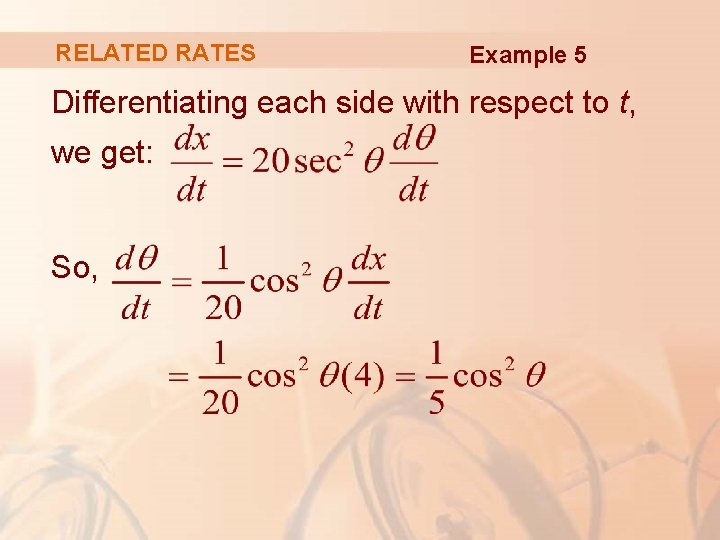RELATED RATES Example 5 Differentiating each side with respect to t, we get: So,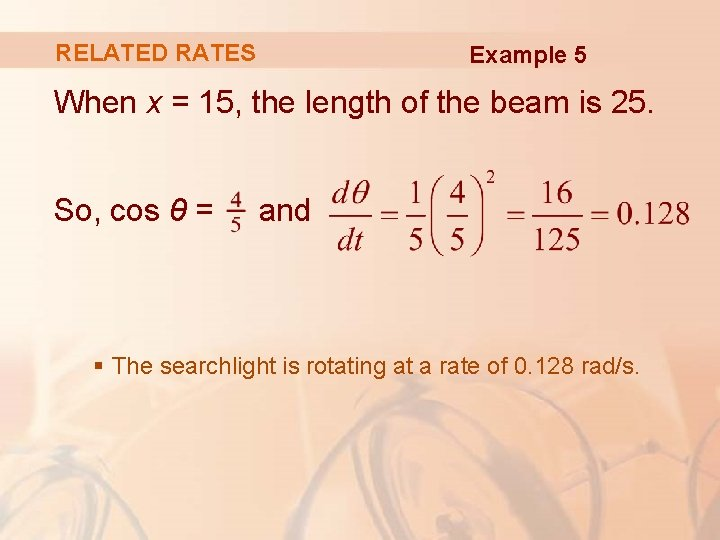RELATED RATES Example 5 When x = 15, the length of the beam is 25. So, cos θ = and § The searchlight is rotating at a rate of 0. 128 rad/s.